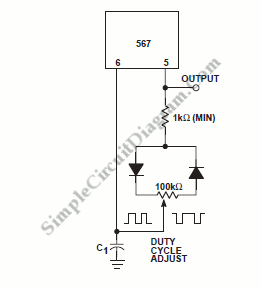# Adjustable Duty Cycle 567 IC Pulse Generator

Pulse generator circuit shown in the schematic diagram below produces square wave pulse with adjustable duty cycle/active factor. The benefits of using this circuit are low components count and high stability of its output frequency.The frequency of this pulse generator is fixed, the frequency f depends on the value of R and C1, following the formula of  f=1/(1.1*R1*C1), where R is the resistance of potentiometer plus 1k fixed resistor. The adjustment of duty cycle (pulse width) doesn’t affect the frequency, since increasing the charging time will decrease the discharging time of C1, compensating the overall period. [Circuit’s schematic diagram source: Philips Semiconductor Application Note]